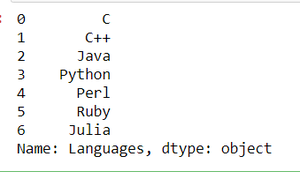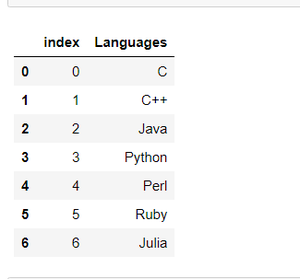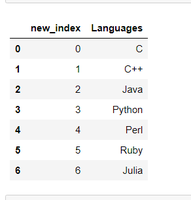GeeksforGeeks App
Open AppBrowser
Continue

# Convert given Pandas series into a dataframe with its index as another column on the dataframe

First of all, let we understand that what are pandas series. Pandas Series are the type of array data structure. It is one dimensional data structure. It is capable of holding data of any type such as string, integer, float etc. A Series can be created using Series constructor.

Syntax: pandas.Series(data, index, dtype, copy)

Return: Series object.

Now, Let’s create a pandas Series:

## Python3

 `# importing pandas package``import` `pandas as pd`` ` `# Creating Series of ``# programming languages``s ``=` `pd.Series([``'C'``, ``'C++'``, ``'Java'``, ``               ``'Python'``, ``'Perl'``, ``'Ruby'``,``               ``'Julia'``])`` ` `s`

Output:Now, we will convert given Pandas series into a dataframe with its index as another column on the dataframe by using Series.to_frame() and Dataframe.reset_index() methods together.

Syntax: Series.to_frame(name=None)

Return: Dataframe.

Syntax: Dataframe.reset_index(level=None, drop=False, name=None, inplace=False)

Return: Dataframe.

Example 1: We will convert given Pandas series into a dataframe with its index as another column.

## Python3

 `# using series.to_frame to``# convert series to dataframe``df ``=` `s.to_frame().reset_index()`` ` `# show the dataframe``df`

Output:Example 2: We can also rename our index column.

## Python3

 `# Renaming our index column as 'new_index'``df.rename(columns ``=` `{``'index'``:``'new_index'``},``          ``inplace ``=` `True``)`` ` `# show the dataframe``df`

Output:My Personal Notes arrow_drop_up